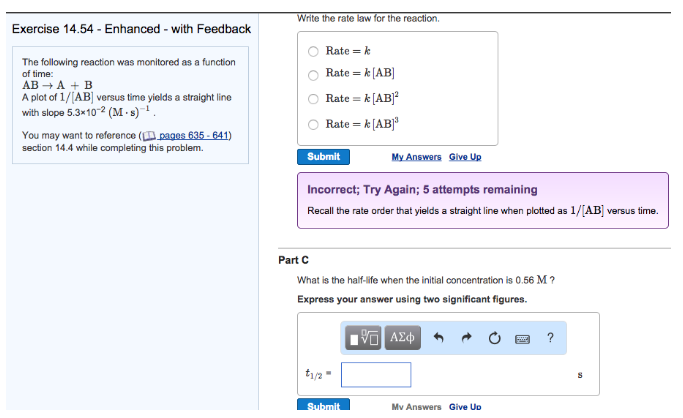# Problem: The following reaction was monitored as a function of time: AB → A + B A plot of 1/[AB] versus time yields a straight line with slope 5.3 x 10 -2 (M • s)-1. Write the rate law for the reaction. What is the half-life when the initial concentration is 0.56 M? Express your answer using two significant figures.

###### FREE Expert Solution
96% (260 ratings)###### Problem Details

The following reaction was monitored as a function of time:

AB → A + B

A plot of 1/[AB] versus time yields a straight line with slope 5.3 x 10 -2 (M • s)-1

Write the rate law for the reaction.

What is the half-life when the initial concentration is 0.56 M? Express your answer using two significant figures.Frequently Asked Questions

What scientific concept do you need to know in order to solve this problem?

Our tutors have indicated that to solve this problem you will need to apply the Integrated Rate Law concept. You can view video lessons to learn Integrated Rate Law. Or if you need more Integrated Rate Law practice, you can also practice Integrated Rate Law practice problems.